# 9 (number)

 9 0 1 2 3 4 5 6 7 8 9 >> List of numbers — Integers 0 10 20 30 40 50 60 70 80 90 >> Cardinal 9 nine Ordinal 9th ninth Numeral system novenary Factorization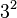$3^2$ Divisors 1, 3, 9 Amharic ፱ Roman numeral IX Roman numeral (Unicode) Ⅸ, ⅸ prefixes ennea- (from Greek) nona- (from Latin) Binary 1001 Octal 11 Duodecimal 9 Hexadecimal 9 Arabic-Indic numeral ٩ Armenian numeral Թ Bengali ৯ Chinese/Japanese numeral 九 玖 (formal writing) Devanāgarī ९ Greek numeral θ´ Hebrew numeral ט (Tet) Tamil numeral ௯ Khmer ៩ Thai numeral ๙

9 (nine) is a number, numeral, and glyph that represents the number. It is the natural number that follows 8 and precedes 10. It is an integer and a cardinal number, that is, a number that is used for counting. In addition, it is classified as a real number, distinguishing it from imaginary numbers. Nine is the highest single-digit number in the decimal system. A group of nine is called an ennead.

## Evolution of the glyph

According to Georges Ifrah, the origin of the 9 integers can be traced to the ancient Indian civilization. These numbers were adopted by subsequent civilizations in conjunction with the 0.At first, various Indians wrote 9 similar to the modern closing question mark without the bottom dot. The Kshtrapa, Andhra, and Gupta started curving the bottom vertical line coming up with a 3 look-alike. The Nagari continued the bottom stroke to make a circle and enclose the 3 look-alike, in much the same way that the @ character encircles a lowercase a. Over time, the enclosing circle became bigger and its line continued beyond the circle downwards, as the 3 look-alike became smaller. Soon, all that was left of the 3 look-alike was a squiggle. The Arabs simply connected that squiggle to the downward stroke at the middle, and subsequent European change was purely cosmetic.

While the shape of the 9 character has an ascender in most modern typefaces, in typefaces with text figures the character usually has a descender, for example, in.

This numeral resembles an inverted 6. To disambiguate the two on objects and documents that could be inverted, the 9 has often been underlined (as is done for the 6). Another distinction from the 6 is that 9 is often handwritten with a straight stem.

## In mathematics

Nine is a composite number, its proper divisors being 1 and 3. It is 3 times 3 and hence the third square number. 9 is a Motzkin number. It is the first composite lucky number.

9 is the second non-unitary square prime (32). It has a unique aliquot sum 4 which is itself a square prime. 9 is the only square prime with an aliquot sum of the same form. The aliquot sequence of 9 has 5 members (9,4,3,1,0) this number being the second composite member of the 3-aliquot tree.

There are nine Heegner numbers.

Since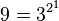$9 = 3^{2^1}$, 9 is an exponential factorial.

Eight and 9 form a Ruth-Aaron pair under the second definition that counts repeated prime factors as often as they occur.

A polygon with nine sides is called an enneagon (technically) or nonagon (in common usage). A group of nine of anything is called an ennead.

In base 10, a number is evenly divisible by nine if and only if its digital root is 9. That is, if you multiply nine by any whole number (except zero), and repeatedly add the digits of the answer until it's just one digit, you will end up with nine:

• 2 × 9 = 18 (1 + 8 = 9)
• 3 × 9 = 27 (2 + 7 = 9)
• 9 × 9 = 81 (8 + 1 = 9)
• 121 × 9 = 1089 (1 + 0 + 8 + 9 = 18; 1 + 8 = 9)
• 234 × 9 = 2106 (2 + 1 + 0 + 6 = 9)
• 578329 × 9 = 5204961 (5 + 2 + 0 + 4 + 9 + 6 + 1 = 27 (2 + 7 = 9))
• 482729235601 × 9 = 4344563120409 (4 + 3 + 4 + 4 + 5 + 6 + 3 + 1 + 2 + 0 + 4 + 0 + 9 = 45 (4 + 5 = 9))
• (Exception) 0 x 9 = 0 (0 is not equal to 9)(though it can be considered -1 in the tens place and 10 in the ones place(10-1=9))

The only other number with this property is three. In base N, the divisors of N − 1 have this property. Another consequence of 9 being 10 − 1, is that it is also a Kaprekar number.

The difference between a base-10 positive integer and the sum of its digits is a whole multiple of nine. Examples:

• The sum of the digits of 41 is 5, and 41-5 = 36. The digital root of 36 is 3+6 = 9, which, as explained above, demonstrates that it is evenly divisible by nine.
• The sum of the digits of 35967930 is 3+5+9+6+7+9+3+0 = 42, and 35967930-42 = 35967888. The digital root of 35967888 is 3+5+9+6+7+8+8+8 = 54, 5+4 = 9.

Subtracting two base-10 positive integers that are transpositions of each other yields a number that is a whole multiple of nine. Some examples:

• 41-14 = 27. The digital root of 27 is 2+7 = 9.
• 36957930-35967930 = 990000, which is obviously a multiple of nine.

This works regardless of the number of digits that are transposed. For example, the largest transposition of 35967930 is 99765330 (all digits in descending order) and its smallest transposition is 03356799 (all digits in ascending order); subtracting pairs of these numbers produces:

• 99765330-35967930 = 63797400; 6+3+7+9+7+4+0+0 = 36, 3+6 = 9.
• 99765330-03356799 = 96408531; 9+6+4+0+8+5+3+1 = 36.
• 35967930-03356799 = 32611131; 3+2+6+1+1+1+3+1 = 18, 1+8 = 9.

Casting out nines is a quick way of testing the calculations of sums, differences, products, and quotients of integers, known as long ago as the twelfth century.

Every prime in a Cunningham chain of the first kind with a length of 4 or greater is congruent to 9 mod 10 (the only exception being the chain 2, 5, 11, 23, 47).

Six recurring nines appear in the decimal places 762 through 767 of pi. This is known as the Feynman point.

If an odd perfect number is of the form 36k + 9, it has at least nine distinct prime factors.

Nine is the binary complement of number six:

9 = 1001
6 = 0110


### In numeral systems

Base Numeral system
2 binary 1001
3 ternary 100
4 quaternary 21
5 quinary 14
6 senary 13
7 septenary 12
8 octal 11
9 novenary 10

### List of basic calculations

Multiplication 1 2 3 4 5 6 7 8 9 10 11 12 13 14 15 16 17 18 19 20 21 22 23 24 25 50 100 1000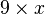$9 \times x$ 9 18 27 36 45 54 63 72 81 90 99 108 117 126 135 144 153 162 171 180 189 198 207 216 225 450 900 9000
Division 1 2 3 4 5 6 7 8 9 10 11 12 13 14 15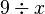$9 \div x$ 9 4.5 3 2.25 1.6 1.5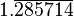$1.\overline{285714}$ 1.125 1 0.9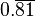$0.\overline{81}$ 0.75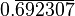$0.\overline{692307}$$0.6\overline{428571}$ 0.6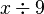$x \div 9$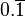$0.\overline{1}$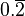$0.\overline{2}$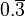$0.\overline{3}$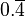$0.\overline{4}$$0.\overline{5}$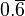$0.\overline{6}$$0.\overline{7}$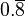$0.\overline{8}$ 1$1.\overline{1}$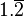$1.\overline{2}$$1.\overline{3}$$1.\overline{4}$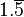$1.\overline{5}$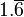$1.\overline{6}$
Exponentiation 1 2 3 4 5 6 7 8 9 10 11 12 13$9 ^ x\,$ 9 81 729 6561 59049 531441 4782969 43046721 387420489 3486784401 31381059609 282429536481 2541865828329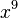$x ^ 9\,$ 1 512 19683 262144 1953125 10077696 40353607 134217728 387420489 1000000000 2357947691 5159780352 10604499373
Radix 1 5 10 15 20 25 30 40 50 60 70 80 90 100
110 120 130 140 150 200 250 500 1000 10000 100000 1000000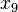$x_{9} \$ 1 5$11_{9} \$$16_{9} \$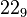$22_{9} \$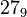$27_{9} \$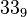$33_{9} \$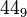$44_{9} \$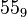$55_{9} \$$66_{9} \$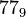$77_{9} \$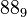$88_{9} \$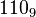$110_{9} \$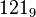$121_{9} \$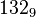$132_{9} \$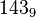$143_{9} \$$154_{9} \$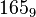$165_{9} \$$176_{9} \$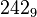$242_{9} \$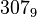$307_{9} \$$615_{9} \$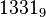$1331_{9} \$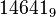$14641_{9} \$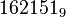$162151_{9} \$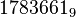$1783661_{9} \$

## In science

### Biology

• A human pregnancy normally lasts nine months.

### Astronomy

• Before 2006 (when Pluto was officially designated a dwarf planet), the Solar System was said to have nine planets.
• The Saros number of the solar eclipse series that began on February 6, 2568 B.C.E. and ended on April 4, 1252 B.C.E. The duration of Saros series 9 was 1316.20 years, and it contained 74 solar eclipses.
• The Saros number of the lunar eclipse series that began on June 26, 2501 B.C.E. and ended on September 5, 1167 B.C.E. The duration of Saros series 9 was 1334.23 years, and it contained 75 lunar eclipses.

### Probability

• In probability calculations, the nine is a logarithmic measure of probability of an event, defined as the negative of the base-10 logarithm of the probability of the event's complement. For example, an event that is 99 percent likely to occur has an unlikelihood of 1 percent or 0.01, which amounts to −log10 0.01 = 2 nines of probability.
• Zero probability gives zero nines (−log10 1 = 0).
• The purity of chemicals, the effectivity of processes, the availability of systems, and so on, can similarly be expressed in nines. For example, "five nines" (99.999 percent) availability implies a total downtime of no more than five minutes per year.

## In technology

• ISO 9 is the standard of the International Organization for Standardization for the transliteration of Cyrillic characters into Latin characters.
• In the Rich Text Format specification, 9 is the language code for the English language.
• In computer system administration, uptime (a measure of the time a computer system has been up and running) is sometimes measured in "nines"—that is, the number of "9" digits in the percentage of time the computer is normally running. For example, "five nines" means 99.999 percent reliability, which translates to a total downtime of no longer than five minutes per year.

## In religion

### Judaism

• The first nine days of the Hebrew month of Av are collectively known as "The Nine Days" (Tisha HaYamim), and are a period of semi-mourning leading up to Tisha B'Av, the ninth day of Av on which both Temples in Jerusalem were destroyed.

### Christianity

• Jesus healed ten lepers, nine of whom did not even thank him.
• In the Catholic Church, a novena is a devotion consisting of prayers said (most typically) on nine successive days, asking to obtain special graces.
• In the Catholic faith, there are 9 choirs of holy angels.

### Bahá'í

• Nine, as the highest single-digit number (in base ten), symbolizes completeness in the Bahá'í Faith.
• The word Bahá' in the Abjad notation has a value of 9.
• A 9-pointed star is used to symbolize the Bahá'í religion.

### Buddhism

• Important Buddhist rituals usually involve nine monks.

## As lucky or unlucky number, Chinese culture

Nine (九 pinyin jiǔ) is considered a good number in Chinese culture because it sounds the same as the word "longlasting" (久 pinyin jiǔ). The Japanese consider 9 to be unlucky, however, because it sounds similar to the Japanese word for "pain" or "distress" (苦 kunrei ku). Fear of the number nine is called enneaphobia.

Nine is strongly associated with the Chinese dragon, a symbol of magic and power. There are nine forms of the dragon, it is described in terms of nine attributes, and it has nine children. It has 9×13 scales, 9×9 being yang (masculine, or bad influence) and 9×4 being yin (feminine, or good influence).

The dragon often symbolizes the Emperor, and the number nine can be found in many ornaments in the Forbidden City.

The circular altar platform (Earthly Mount) of the Temple of Heaven has one circular marble plate in the center, surrounded by a ring of nine plates, then by a ring of 18 plates, and so on, for a total of nine rings, with the outermost having 81=9×9 plates.

## In music

• In music theory, a ninth is the ninth note of a musical scale or the interval between the first note and the ninth. A ninth chord is a chord with a ninth.
• In Western classical music, the "curse of the ninth" refers to the superstition that a composer who writes a ninth symphony will die soon. Beethoven, who left his Tenth Symphony unfinished, is regarded by the superstition as the first victim of the curse.
• A group of nine voices or instruments is called a nonet.

## In sports

• Nine ball is the standard professional pocket billiards variant played in the United States.
• In association football (soccer), the center-forward/striker traditionally (since at least the fifties) wears the number 9 shirt.
• In baseball, nine represents the right fielder's position.
• In rugby union, nine is the number generally worn by the scrum half.
• In rugby league, nine is the number generally worn by the hooker.

## In other fields

• The Enneagram is one system of knowledge which shows the correspondence between the 9 integers and the circle.
• In the NATO phonetic alphabet, the digit 9 is called "Novenine."
• Nine judges sit on the United States Supreme Court.
• The word "K-9" pronounces the same as canine and is used in many U.S. police departments to denote the unit working with police dog.
• There are nine circles of Hell in Dante's "Divine Comedy."
• Stanines are measured on a scale of 1 to 9.
• Nine is a significant number in Norse Mythology. Odin hung himself on an ash tree for nine days to learn the runes.
• The name of the area called Kowloon in Hong Kong literally means: Nine dragons.
• Someone dressed "to the nines" is dressed up as much as they can be.
• There is a saying that a cat has nine lives.
• The Nine Worthies were nine historical, scriptural, mythological or semi-legendary figures who, in the Middle Ages, were believed to personify the ideals of chivalry.
• Nine Unknown Men were, in occult legend, custodians of the sciences of the world since ancient times.
• Many mathematical magic tricks have a connection to the number nine, that because every multiplication of nine adds up to nine, any number minus the sum of all digits becomes a multiplication of nine, and any three digit number minus the same number in reverse becomes a multiplication of nine.